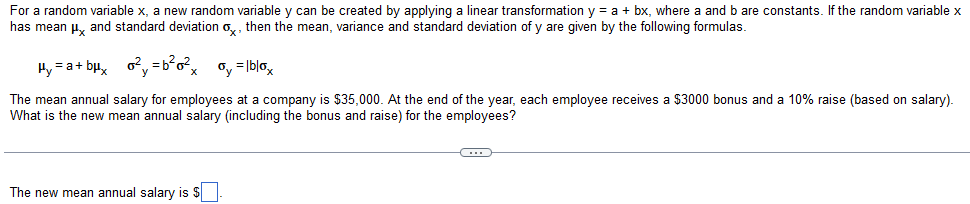Home / Expert Answers / Algebra / for-a-random-variable-x-a-new-random-variable-y-can-be-created-by-applying-a-linear-transformatio-pa114

# (Solved): For a random variable x, a new random variable y can be created by applying a linear transformatio ...For a random variable , a new random variable can be created by applying a linear transformation , where and are constants. If the random variable has mean and standard deviation , then the mean, variance and standard deviation of are given by the following formulas. The mean annual salary for employees at a company is . At the end of the year, each employee receives a bonus and a raise (based on salary). What is the new mean annual salary (including the bonus and raise) for the employees? The new mean annual salary is

We have an Answer from Expert